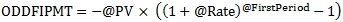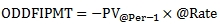# SQL Server interest payment odd-first period annuities

ODDFIPMT

Updated: 16 November 2014

Use the scalar function ODDFIPMT to calculate the interest portion of a periodic payment for an annuity where the first period is either longer or shorter than the other periods. The interest for the first period is calculated as:ElseSyntax
SELECT [wct].[ODDFIPMT](
<@Rate, float,>
,<@Per, int,>
,<@Nper, int,>
,<@PV, float,>
,<@FV, float,>
,<@FirstPeriod, float,>)
Arguments
@Rate
the periodic interest rate. @Rate is an expression of type float or of a type that can be implicitly converted to float.
@Per
the period of interest. @Per is an expression of type int or of a type that can be implicitly converted to int.
@Nper
the number of annuity payments. @Nper is an expression of type int or of a type that can be implicitly converted to int.
@PV
the present value of the annuity. @PV is an expression of type float or of a type that can be implicitly converted to float.
@FV
the future value as at the end of the annuity. @FV is an expression of type float or of a type that can be implicitly converted to float.
@FirstPeriod
the length of the first period. @FirstPeriod is an expression of type float or of a type that can be implicitly converted to float.
Return Type
float
Remarks
·         If @Rate <= -1 then NULL is returned.
·         If @Nper < 1 then NULL is returned.
·         If @FirstPeriod <= 0 then NULL is returned.
·         If @Per < 1 Then NULL is returned.
·         If @Per > @Nper Then NULL is returned.
·         If @Nper is NULL then @Nper = 0.
·         If @Rate is NULL then @Rate = 0.
·         If @PV is NULL then @PV = 0.
·         If @FV is NULL then @FV = 0.
·         If @Per is NULL then @Per = 0.
·         If @FirstPeriod is NULL then @FirstPeriod = 1.
·         ODDFIPMT uses the same conventions for the sign of the inputs and the results as Excel and Google spreadsheets; generally @PV and @FV should have opposite signs and the ODDFPMT result will have the opposite sign of @PV.
Examples
Calculate the interest portion for the first period of an annuity assuming a periodic rate of 0.5%, with 36 periodic payments. The price of the annuity is 11,500 and there is no cash value at the end of the annuity. The first period is 1 and 5/6th longer than the other periods.
SELECT
wct.ODDFIPMT(
.005      --@Rate
,1         --@Per
,36        --@Nper
,-11500     --@PV
,0         --@FV
,1+5/6e+00 --@FirstPeriod
) as IPMT
This produces the following result.
IPMT
----------------------
105.636223805979

Using the same basic information in this SQL, we calculate the periodic interest for each of the first 10 payments.
SELECT
x.Per
,wct.ODDFIPMT(
.005      --@Rate
,x.Per     --@Per
,36        --@Nper
,-11500    --@PV
,0         --@FV
,1+5/6e+00 --@FirstPeriod
) as IPMT
FROM (
VALUES (1),(2),(3),(4),(5),(6),(7),(8),(9),(10)
)x(Per)
This produces the following result.
Per         IPMT
----------- ----------------------
1           105.636223805979
2           56.2716341574074
3           54.7964453665719
4           53.3138806317822
5           51.8239030733186
6           50.3264756270627
7           48.8215610435755
8           47.3091218871708
9           45.7891205349841
10          44.2615191760365

Calculate the interest portion of the first periodic payment for an annuity assuming a periodic rate of 0.5%, with 180 periodic payments. The price of the annuity is 250,000 and there is a 50,000 cash value at the end of the annuity. The first period is one-half as long as the other periods.
SELECT
wct.ODDFIPMT(
.005     --@Rate
,1        --@Per
,180      --@Nper
,-250000  --@PV
,50000    --@FV
,0.5      --@FirstPeriod
) as IPMT
This produces the following result.
IPMT
----------------------
624.220697042766

Using the same basic information in this SQL, we calculate the periodic interest for each of the last 10 payments.
SELECT
x.Per
,wct.ODDFIPMT(
.005         --@Rate
,x.Per        --@Per
,180          --@Nper
,-250000      --@PV
,50000        --@FV
,0.5          --@FirstPeriod
) as IPMT
FROM (
VALUES (171),(172),(173),(174),(175),(176),(177),(178),(179),(180)
)x(Per)
This produces the following result.
Per         IPMT
----------- ----------------------
171         331.855107050157
172         323.852086351572
173         315.809050549495
174         307.725799568407
175         299.602132332414
176         291.437846760241
177         283.232739760207
178         274.986607225173
179         266.699244027464
180         258.370444013766

Calculate the interest portion of the first weekly payment for an automobile lease with a term of 3 years and an annual interest rate of 25%. The amount to be financed is 11,000 and the residual value at the end of the lease is 3,500. The first payment is due 2014-11-25.
SELECT
wct.ODDFIPMT(
.25 * 7/365e+00     --@Rate
,1                   --@Per
,156                 --@Nper
,-11000              --@PV
,3500                --@FV
,DATEDIFF(d,'2014-11-13','2014-11-25')/7e+00    --@FirstPeriod
) as IPMT
This produces the following result.
IPMT
----------------------
90.5657016076089

Using the same basic information in this SQL, we calculate the periodic interest for each of the first 10 payments.
SELECT
x.Per
,wct.ODDFIPMT(
.25 * 7/365e+00     --@Rate
,x.Per               --@Per
,156                 --@Nper
,-11000              --@PV
,3500                --@FV
,DATEDIFF(d,'2014-11-13','2014-11-25')/7e+00    --@FirstPeriod
) as IPMT
FROM (
VALUES (1),(2),(3),(4),(5),(6),(7),(8),(9),(10)
)x(Per)
This produces the following result.
Per         IPMT
----------- ----------------------
1           90.5657016076089
2           52.7639606027343
3           52.6069539540754
4           52.4491945338133
5           52.2906787327692
6           52.1314029244598
7           51.9713634650146
8           51.8105566930927
9           51.6489789297985
10          51.4866264785981

### SupportCopyright 2008-2021 Westclintech LLC         Privacy Policy        Terms of Service